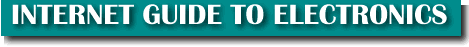OHMS LAW
EXPLAINED
The amount of current flowing in a circuit made up of pure resistances is directly proportional to the electromotive forces impressed on the circuit and inversely proportional to the total resistance of the circuit.

Don't let that quote scare you.  It is not as scholarly as it sounds.

 In simpler terms, Ohms Law means:  1) A steady increase in voltage, in a circuit with constant resistance, produces a constant linear rise in current.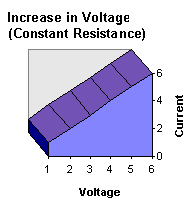2) A steady increase in resistance, in a circuit with constant voltage, produces a progressively (not a straight-line if graphed) weaker current.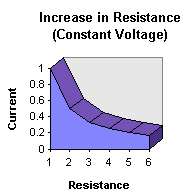Ohms Law is a set of formulas used in electronics to calculate an unknown amount of current, voltage or reistance.  It was named after the German physicist Georg Simon Ohm. Born 1787.  Died 1854.

Knowledge of this Law is often under-estimated by beginners.  I have talked to people that can design complex circuitry and microprocessor systems that have said, Ohms Law?  What's that?

Unless you know this basic fundamental building block of electronics, you will never have a strong foundation to hold up the electronics towers you will be constructing in the future.  Learn Ohms Law.  Learn it inside and out!

Ohm's Law is a formulation of the relationship of voltage, current, and resistance, expressed as: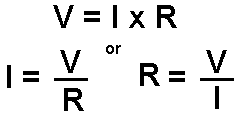Where:
V is the Voltage measured in volts
I is the Current measured in amperes
R is the resistance measured in Ohms

Therefore:
Volts = Amps times Resistance

Ohms Law is used to calculate a missing value in a circuit.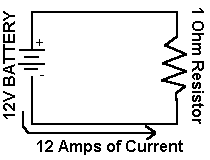In this simple circuit there is a current of 12 amps (12A) and a resistive load of 1 Ohm (1W).  Using the first formula from above we determine the Voltage:
V = 12 x 1 : V = 12 Volts (12V)

If we knew the battery was supplying 12 volt of pressure (voltage), and there was a resistive load of 1 Ohm placed in series, the current would be:

I = 12 / 1 : I = 12 Amps (12A)

If we knew the battery was supplying 12V and the current being generated was 12A, then the Resistance would be:

R = 12/12 : R = 1W

 Note: Remember a battery is not measured in amperage as is commonly believed with beginners to electronics.  The battery supplies the pressure that creates the flow (current) in a given circuit.  The amperage rating on a battery is "How long the battery will last for one hour while driving a circuit of that amperage".  It is measured in Amperage-Hours.  So a 1000mAh would last for 1 hour in a one amp circuit. (1000mAh is 1A for one hour)
An easy way to remember the formulas is by using this diagram.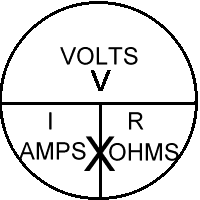To determine a missing value, cover it with your finger.  The horizontal line in the middle means to divide the two remaining values.  The "X" in the bottom section of the circle means to multiply the remaining values.

If you are calculating voltage, cover it and you have I X R left (V= I times R).

If you are calculating amperage, cover it, and you have V divided by R left (I=V/R).

If you are calculating resistance, cover it, and you have V divide by I left (R=V/I).

Note: The letter E is sometimes used instead of V for voltage.

TOP